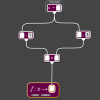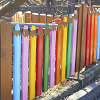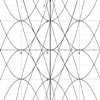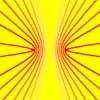# Resources tagged with: Quadratic functions and graphs

Filter by: Content type:
Age range:
Challenge level:

There are 17 NRICH Mathematical resources connected to Quadratic functions and graphs, you may find related items under Coordinates, Functions and Graphs.

Broad Topics > Coordinates, Functions and Graphs > Quadratic functions and graphs##### Age 14 to 16Challenge Level

Explore the relationship between quadratic functions and their graphs.### Minus One Two Three

##### Age 14 to 16Challenge Level

Substitute -1, -2 or -3, into an algebraic expression and you'll get three results. Is it possible to tell in advance which of those three will be the largest ?### Spaces for Exploration

##### Age 11 to 14

Alf Coles writes about how he tries to create 'spaces for exploration' for the students in his classrooms.### Fence It

##### Age 11 to 14Challenge Level

If you have only 40 metres of fencing available, what is the maximum area of land you can fence off?### Parabolas Again

##### Age 14 to 18Challenge Level

Here is a pattern composed of the graphs of 14 parabolas. Can you find their equations?### Parabella

##### Age 16 to 18Challenge Level

This is a beautiful result involving a parabola and parallels.### Parabolic Patterns

##### Age 14 to 18Challenge Level

The illustration shows the graphs of fifteen functions. Two of them have equations y=x^2 and y=-(x-4)^2. Find the equations of all the other graphs.### Guessing the Graph

##### Age 14 to 16Challenge Level

Can you suggest a curve to fit some experimental data? Can you work out where the data might have come from?##### Age 14 to 18Challenge Level

This task develops knowledge of transformation of graphs. By framing and asking questions a member of the team has to find out which mathematical function they have chosen.### Geometric Parabola

##### Age 14 to 16Challenge Level

Explore what happens when you draw graphs of quadratic equations with coefficients based on a geometric sequence.### ' Tis Whole

##### Age 14 to 18Challenge Level

Take a few whole numbers away from a triangle number. If you know the mean of the remaining numbers can you find the triangle number and which numbers were removed?### Integral Inequality

##### Age 16 to 18Challenge Level

An inequality involving integrals of squares of functions.### More Parabolic Patterns

##### Age 14 to 18Challenge Level

The illustration shows the graphs of twelve functions. Three of them have equations y=x^2, x=y^2 and x=-y^2+2. Find the equations of all the other graphs.### Consecutive Squares

##### Age 14 to 16Challenge Level

The squares of any 8 consecutive numbers can be arranged into two sets of four numbers with the same sum. True of false?### Converse

##### Age 14 to 16Challenge Level

Clearly if a, b and c are the lengths of the sides of an equilateral triangle then a^2 + b^2 + c^2 = ab + bc + ca. Is the converse true?### Grid Points on Hyperbolas

##### Age 16 to 18Challenge Level

Find a condition which determines whether the hyperbola y^2 - x^2 = k contains any points with integer coordinates.